# 神经网络Python实现（9行代码）

1. 神经网络简介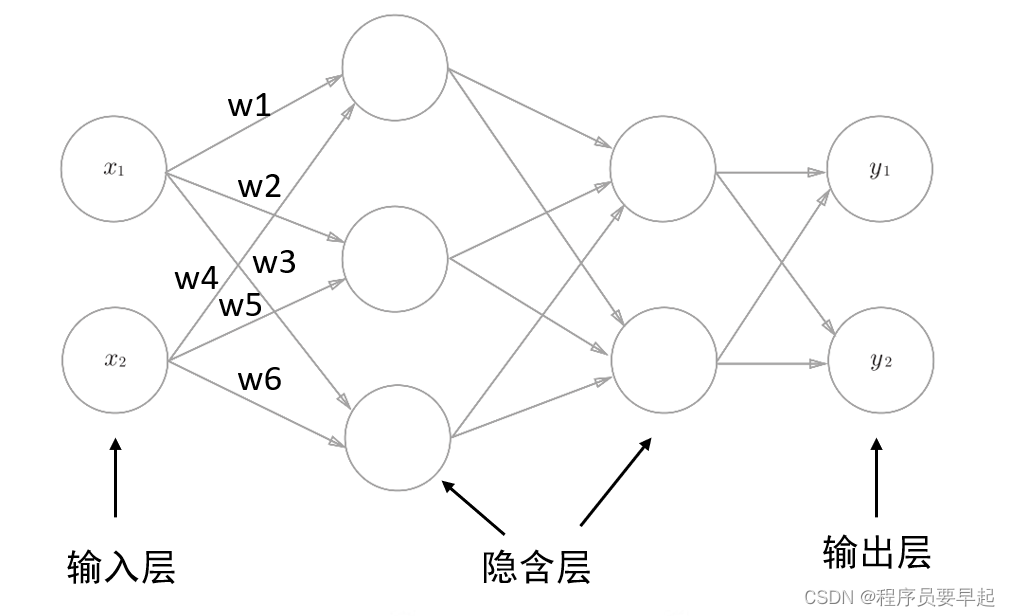下面介绍一下单个神经元：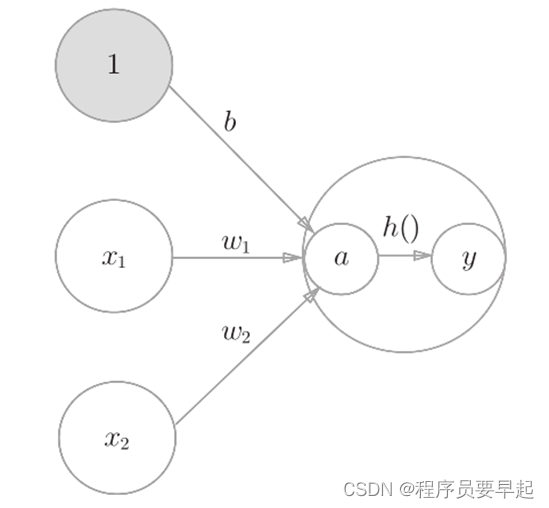a = x1*w1 + x2*w2 + b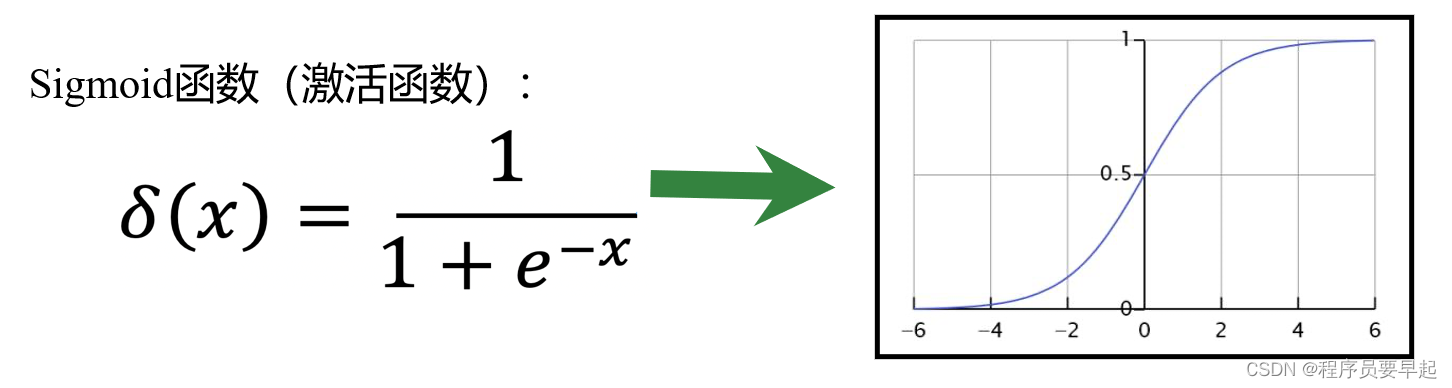2. 代码解释

https://github.com/miloharper/simple-neural-network

A neural network written in Python, consisting of a single neuron that uses gradient descent to learn.

``````from numpy import exp, array, random, dot
training_set_inputs = array([[0, 0, 1], [1, 1, 1], [1, 0, 1], [0, 1, 1]])
training_set_outputs = array([[0, 1, 1, 0]]).T
random.seed(1)
synaptic_weights = 2 * random.random((3, 1)) - 1
for iteration in range(10000):
output = 1 / (1 + exp(-(dot(training_set_inputs, synaptic_weights))))
synaptic_weights += dot(training_set_inputs.T, (training_set_outputs - output) * output * (1 - output))
print( 1 / (1 + exp(-(dot(array([1, 0, 0]), synaptic_weights)))))``````

from numpy import exp, array, random, dot

#从Numpy库中调用exp（指数函数）、array（数组）、random（随机函数）、dot（矩阵相乘函数）

training_set_inputs = array([[0, 0, 1], [1, 1, 1], [1, 0, 1], [0, 1, 1]])

#神经网络训练部分的输入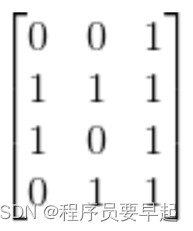training_set_outputs = array([[0, 1, 1, 0]]).T

#神经网络训练部分的输出，.T表示矩阵转置random.seed(1)

#使用随机函数生成随机数（这一行代码可以省略，目的只是保证测试结果与作者一致）

synaptic_weights = 2 * random.random((3, 1)) 1for iteration in range(10000):
output = 1 / (1 + exp(-(dot(training_set_inputs, synaptic_weights))))

#使用for语句循环10000次，将训练集的输入和权重采用.dot进行矩阵相乘，将相乘得到的结果输入到sigmoid函数，然后将得到的结果赋值给output

synaptic_weights += dot(training_set_inputs.T, (training_set_outputs – output) * output * (1 – output))

#权重的调整采用“误差加权导数”公式（梯度下降）print (1 / (1 + exp(-(dot(array([1, 0, 0]), synaptic_weights))))) #[0.99993704]

#synaptic_weights是调整之后的最终权重，数组（矩阵）[1，0，0]与这个权重矩阵通过dot函数进行相乘，将相乘的结果作为输入引入到sigmoid函数，得到最终的结果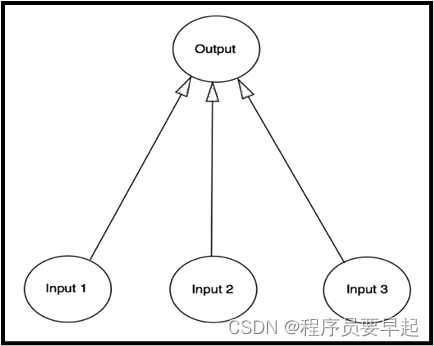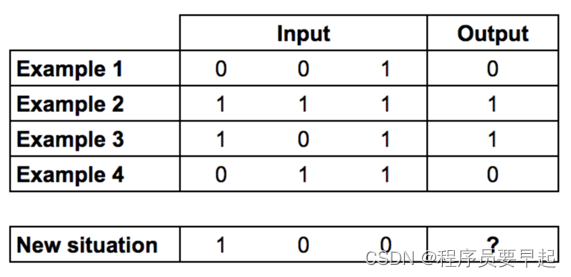这9行代码就是把上图的1~4组数据作为训练集进行模型训练，不断地更新权重使其输出更接近训练集中给出的输出标签（标准答案），然后将最后一组数据当做测试集来检测模型的准确度，它最后的结果输出是0.99993704，也是约等于1，其实不难发现，4组测试数据的输出都与第一个输入数据相同，所以说神经网络模型测试得到了正确的结果，这也是说明神经网络有预测结果的作用。

(0)